# Understanding Process Sigma Level

By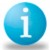Mahmoud Razzaghi

Six Sigma is a data-driven approach to quality, aimed at reducing variation and the associated defects, wastes and risks in any process. This article explores the basics of Six Sigma process quality – definition and measurement.

In a set of data, mean (μ) and standard deviation (σ) are defined as:

μ = x1 + x2 + x3 + … + xn) / n

Where x1 , x2 , , xn are data values and n is the number of data elements, and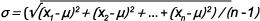Standard deviation shows the extent of variation or spread of data. A larger standard deviation indicates that a data set has a wider spread around its mean. Process data usually has a normal distribution. The distance from the mean μ to a data value in terms of data units can be measured. For example, a data point with a value of x = 31 seconds is 6 seconds away from a mean value of 25 seconds. This distance can also be measured by counting the number of standard deviations in the distance. If the standard deviation is 2 seconds, the same point is 6/2 or 3 standard deviations away from the mean. This count is denoted by sigma level, Z, also known as Z-score, as shown below.
Z = (x – μ) / σ

Z = (31- 25) / 2 = 3

### Specification Limits and Defect Rates

In a process, deviations from the target or mean are accepted to a certain value defined by the specification limits (SL) around the mean. Any value beyond the specification limit indicates a defect or unacceptable result. The farther the specification limits are from the mean, the lower the chance of defects.

Handpicked Content:   Zero Defects: What Does It Achieve? What Does It Mean?

A Six Sigma process has a specification limit which is 6 times its sigma (standard deviation) away from its mean. Therefore, a process data point can be 6 standard deviations from the mean and still be acceptable. (See Figure 1.)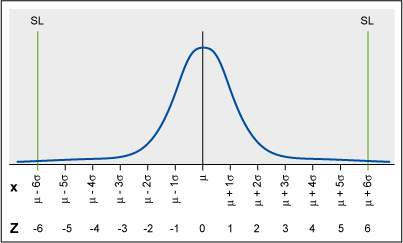Figure 1: Normal Distribution With Mean, Z-score and Six Sigma Specification Limits

In a stable process, the mean naturally shifts as much as 1.5 sigma in the long term on either side of its short-term value. The red lines in Figure 2 (below) show the extreme case of 1.5-sigma mean shift to the right. The right specification limit is at 4.5 sigma from the mean with a defect rate of 3.4 parts per million (PPM). The left specification limit is at 7.5 sigma from the mean with a defect rate of 0 PPM. The overall defect rate, therefore, is 3.4 PPM. A similar argument applies to the extreme case of 1.5-sigma shift to the left. A Six Sigma process is actually 4.5 sigma in the long term, and the 3.4 PPM defect rate is the 1-sided probability of having a data value beyond 4.5 sigma measured from the short-term mean.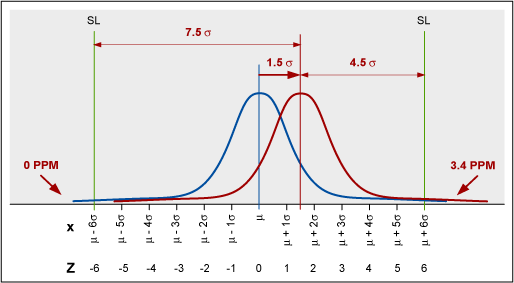Figure 2: Process Mean Shift of 1.5 Sigma and Defect Rate Corresponding to 4.5 Sigma

The 1.5-sigma shift makes defects approach 0 on the opposite side of the shift even at lower sigma levels. The one-sided defect rate is applicable to any capable process with 1-sided or 2-sided SLs, even at a 3-sigma level.

Given the specification limit, SL, the process sigma level, or process Z, is:

Z = (x – μ) / σ = (SL – μ) / σ

In this example, the process sigma level for a specification limit of 31 seconds is:

Handpicked Content:   Yield to Sigma Conversion Table

Z = (SL – μ) / σ

Z  = (31 – 25) / 2 = 3

Therefore, the process is at a 3-sigma quality level. In order to bring the process to the golden Six Sigma quality level, the process sigma would have to be reduced to 1.

Z = (31 – 25) / 1 = 6

In general, the Z formula can be rearranged to calculate the maximum allowable process sigma, or standard deviation, for any sigma level.

Z = (x – μ) / σ

σ = (x – μ ) / Z

For example, given a mean of 25 seconds and SL of 31 seconds, for a Six Sigma quality level, the required process sigma is calculated as:

σ = (31 – 25) / 6 = 1

Similarly, for a 3-sigma quality level, the process sigma must be:

σ = (31 – 25 ) / 3 = 2

Referring back to the short- and long-term behavior of the process mean, there are 2 values for Z, short-term Z, or Zst, and long-term Z, or Zlt.

Zlt = Zst – 1.5

In sigma level calculations, use Zst. A Six Sigma process is 6 sigma in the short term and 4.5 sigma in the long term or:

Zst = 6

Zlt = Zst – 1.5 = 4.5

### Clarifying Process Sigma and Sigma Level

Sometimes the term process sigma is used instead of the process sigma level, which may cause confusion. Process sigma indicates the process variation (i.e., standard deviation) and is measured in terms of data units (such as seconds or millimeters), while process sigma count Z, or process sigma level, is a count with no unit of measure.

### Process Capability and Six Sigma

Another measure of process quality is process capability, or Cp, which is the specification width (distance between the specification limits) divided by 6 times the standard deviation.

Handpicked Content:   Should You Calculate Your Process Sigma?

Cp = (Upper SL – Lower SL) / 6σ

The recommended minimum or acceptable value of Cp is 1.33. In terms of Six Sigma, this process capability is equivalent to a sigma level of 4 and long-term defect rate of 6,210 PPM. Process capability for a Six Sigma process is 2.

##### Mahmoud Razzaghi

Healthcare

1.Kicab Castaneda-Mendez

This is a good explanation of the calculation of sigma scale values for both short and long term. However, we should be moving past this scale for the following reasons.
1. “In a stable process, the mean naturally shifts as much as 1.5 sigma in the long term on either side of its short-term value.” This statement is self-contradictory. A stable process doesn’t shift—by definition. If a stable process could shift that much, then control charts or other tools for assessing stability would be useless as they would need to differentiate between a “natural” shift and “unnatural” shifts. For example, if there is one or more points beyond control charts limits that could come from a shift of the mean less than 1.5 sigma, why does that make the process unstable rather than stable within its natural shift?
2. Six sigma and other process improvement methodologies use facts to make better decisions. There is no factual basis for the 1.5 sigma shift. (If you think there is then provide it.)
3. Sigma merely represents either the percent meeting specifications or the percent not meeting specifications (e.g., DPMO). Hence, there is no need to make the calculations so complicated. Percentages are easily understood while the sigma scale is not—hence, the reason for your article.
4. The calculations you show assume a normal distribution. But since sigma is merely percent meeting specifications, then normality is not required for determining a sigma value. Just determine the percent meeting specs using whatever distributional assumption (e.g., exponential for cycle time) and convert to the sigma scale value. But since you are going to explain the sigma scale as percent meeting spec, the conversion is completely unnecessary.

2.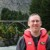Chris Seider

I think this misses a key point. Sigma level on one side amounts to a defective rate. Both defective rates need to be added to get an overall sigma level (which is commonly done in software packages like Minitab).

It can be referred to as Zusl and Zlsl and Zoverall.